Technical Article

# Via Configurations for Connecting Decoupling Capacitors

October 07, 2019 by Dr. Steve Arar

## In this article, we’ll focus on the different via configurations that can be used to connect a decoupling capacitor to the PCB power and ground planes.

The inductance of the loop that connects a decoupling capacitor to a logic IC is of paramount importance and should be minimized. In this article, we’ll focus on the different via configurations that can be used to connect a decoupling capacitor to the PCB power and ground planes. We’ll see that making small changes can significantly reduce the inductance of the decoupling loop.

### Why Do We Need a Decoupling Capacitor?

A clocked digital IC usually needs large transient power supply currents. For example, a large microprocessor can draw currents as large as 10 A in a very short period of time. As the rise/fall time of the IC outputs decreases, we need to provide this transient energy at a higher rate. The power and ground conductors of a PCB do exhibit some inductance. If the large transient current of the digital IC goes through the inductance of the power and ground conductors, there will be a voltage created across the inductance. Due to the large transient voltage drops across the power and ground conductors, we won’t be able to deliver a constant voltage across the power and ground pads of the IC.

The solution to the above problem is to provide a source of charge that can provide the transient currents. This is usually achieved by placing decoupling capacitors very close to each logic IC. We should always keep in mind that the circuit power routing only replenishes the charge in the decoupling capacitor and it is the decoupling capacitor that should supply all the high-frequency transient currents. A decoupling capacitor provides the transient currents in a short time interval associated with the rise/fall time of the IC outputs, and the power supply has at least half a clock cycle to recharge the decoupling capacitors. Keeping the high-frequency energy off the power distribution traces allows us to more easily tolerate the unavoidable inductance of the power distribution structure.

### Inductance of the Decoupling Loop

As discussed above, we use decoupling capacitors to avoid supplying the high-frequency currents through the power distribution traces, which can exhibit a high inductance. That’s why the inductance of the path that connects the decoupling capacitor to the IC is important as well. If the inductance of the decoupling loop is not small enough, the logic IC will try to obtain some of its high-frequency energy through the power distribution structure. Therefore, we need to carefully examine the inductance of the decoupling loop and minimize it in every possible way. Please refer to this article to learn about an important technique for reducing the inductance of the decoupling loop. In this article, we’ll focus on the different via configurations that can be used to connect a decoupling capacitor to the PCB power and ground planes.

### Vias Carrying Currents with Opposite Directions

The conventional method of mounting a decoupling capacitor is placing the vias next to the capacitor pads as shown in Figure 1.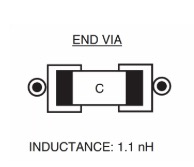##### Figure 1. Image used courtesy of Electromagnetic Compatibility Engineering.

For this case, a typical value for the total inductance from the mounting pads of the capacitor to the power-ground plane pair is about 1.1 nH. To reduce the total inductance of these vias, we can bring them close together. Bringing the vias close together will increase the mutual inductance between them.

Since the currents of these two vias flow in opposite directions, the increased mutual inductance will reduce the net inductance of each via. To better understand this, remember that a current going through an inductor produces magnetic field lines that encircle the conductor. The direction of these magnetic field lines can be found by the right-hand rule shown in Figure 2 (in this example, the current direction is upward).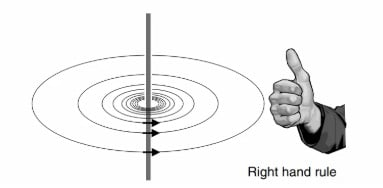##### Figure 2. Image used ƒcourtesy of Signal and Power Integrity-Simplified.

When the current goes through a via, it produces magnetic field lines that encircle it. Some of the magnetic field lines of one via will encircle the other via as well.

Since the two vias produce magnetic fields in the opposite direction (see Figure 3), the magnetic coupling between them will actually reduce the total number of field lines that encircle each via. The number of net magnetic field lines around a conductor determines its inductance. Therefore, when we have vias that carry currents with opposite directions, increasing the magnetic coupling between them will reduce the effective inductance that each via exhibits.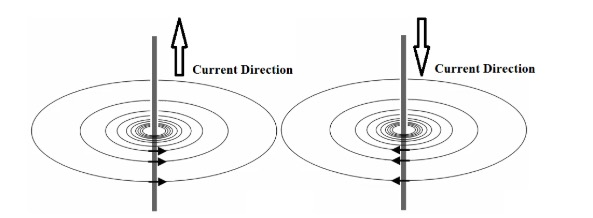##### Figure 3

Therefore, one technique to reduce the inductance of the decoupling loop is to bring the vias close together as shown in Figure 4. In this case, the inductance between the mounting pad of the capacitor and the power-ground plane pair is reduced to 0.7 nH.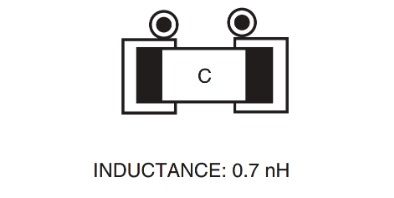### Vias Carrying Currents with the Same Direction

To further reduce the inductance of the decoupling loop, we can use multiple vias rather than using only one via for each capacitor pad. Two possible arrangements are shown in Figure 5.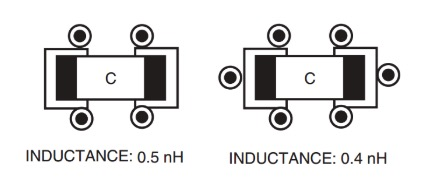##### Figure 5. Image courtesy of Electromagnetic Compatibility Engineering.

If there was no mutual inductance between the vias connected to the same pad, we could easily conclude that the equivalent inductance of the parallel vias will be inversely proportional to the number of vias. What if there is some mutual inductance between them?

The currents through the vias connected to the same pad are in the same direction. Unlike the case in Figure 3, the magnetic coupling between the parallel vias of Figure 5 will increase the total number of field lines that encircle each via. Therefore, when we have vias that carry currents with the same direction, increasing the magnetic coupling between them will increase the effective inductance that each via exhibits.

Let’s consider the arrangement with two vias for each pad (the left figure above). Assume that the self-inductance of each via is L and the mutual inductance between the parallel vias is M (Figure 6 below). What would be the value of the equivalent inductance?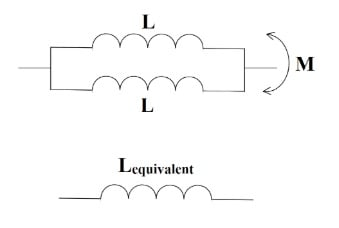##### Figure 6

Considering the fact that the magnetic field lines of the two vias are in the same direction, it can be shown that the equivalent inductance of the two vias is given by:

$L_{equivalent} = \frac {L + M}{2}$

The mutual inductance, M, cannot be greater than the self-inductance L. Therefore, in the worst-case scenario, $L_{equivalent}$ is close to L. However, if we place the vias connected to the same pad sufficiently apart from each other, we can significantly reduce the mutual inductance M and obtain an equivalent inductance close to $\frac{L}{2}$.

It is usually assumed that if the center-to-center spacing between the vias is greater than the length of a via, the mutual inductance becomes much less than the self-inductance. In this case, the equivalent inductance of the vias will be almost inversely proportional to the number of vias.

### Another Advantage of Using Multiple Vias

We saw that, with multiple vias, we can have an equivalent inductance inversely proportional to the number of vias. There is another mechanism that allows the arrangements of Figure 5 to have a lower inductance. To understand this second mechanism, we need to consider the current distribution in a plane when the current is fed into or out of the plane through a via. As shown in Figure 7, in the vicinity of the vias, the current is constricted to flow into or out of the plane through a via. As we move away from the vias, the current can spread out.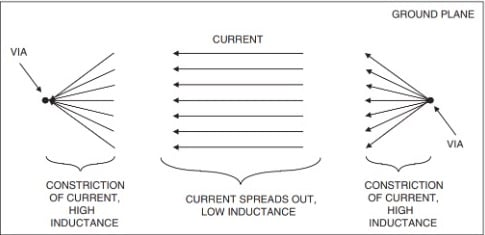##### Figure 7. Image courtesy of Electromagnetic Compatibility Engineering.

Examining the inductance of a plane can be a complicated problem and is beyond the scope of this article. You can find some details in Chapter 10 of the book Electromagnetic Compatibility Engineering. The important point is that away from the vias the current can spread out and the plane exhibits a low inductance. However, near the vias the current cannot spread out and the inductance of the path increases significantly.

Interestingly, the total inductance of the current distribution shown in Figure 7 will be primarily determined by the large inductance in the vicinity of the vias. The arrangements of Figure 5 use several vias to connect to the planes. As a result, the multiple-via configuration allows the current to spread out in a wider area of the plane compared to the configurations that use a single via. Thus, multiple vias can reduce the inductance of the plane in the vicinity of the vias and, consequently, reduce the inductance of the decoupling loop.

### Conclusion

We use decoupling capacitors to avoid supplying high-frequency currents through power distribution traces, which can exhibit high inductance. If the inductance of the decoupling loop is not small enough, the logic IC will try to obtain some of its high-frequency energy through the power distribution structure. Therefore, we need to minimize the inductance of the decoupling loop. In this article, we discussed the different via configurations that can be used to efficiently connect a decoupling capacitor to the PCB power and ground planes. We saw that we should bring vias with opposite-direction currents close together and that with appropriate spacing, a multiple-via configuration can lead to an equivalent via inductance that is inversely proportional to the number of vias. Another advantage of a multiple-via configuration is reducing the plane inductance in the vicinity of the vias.